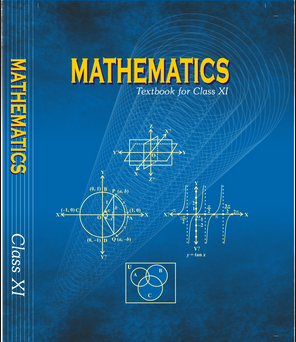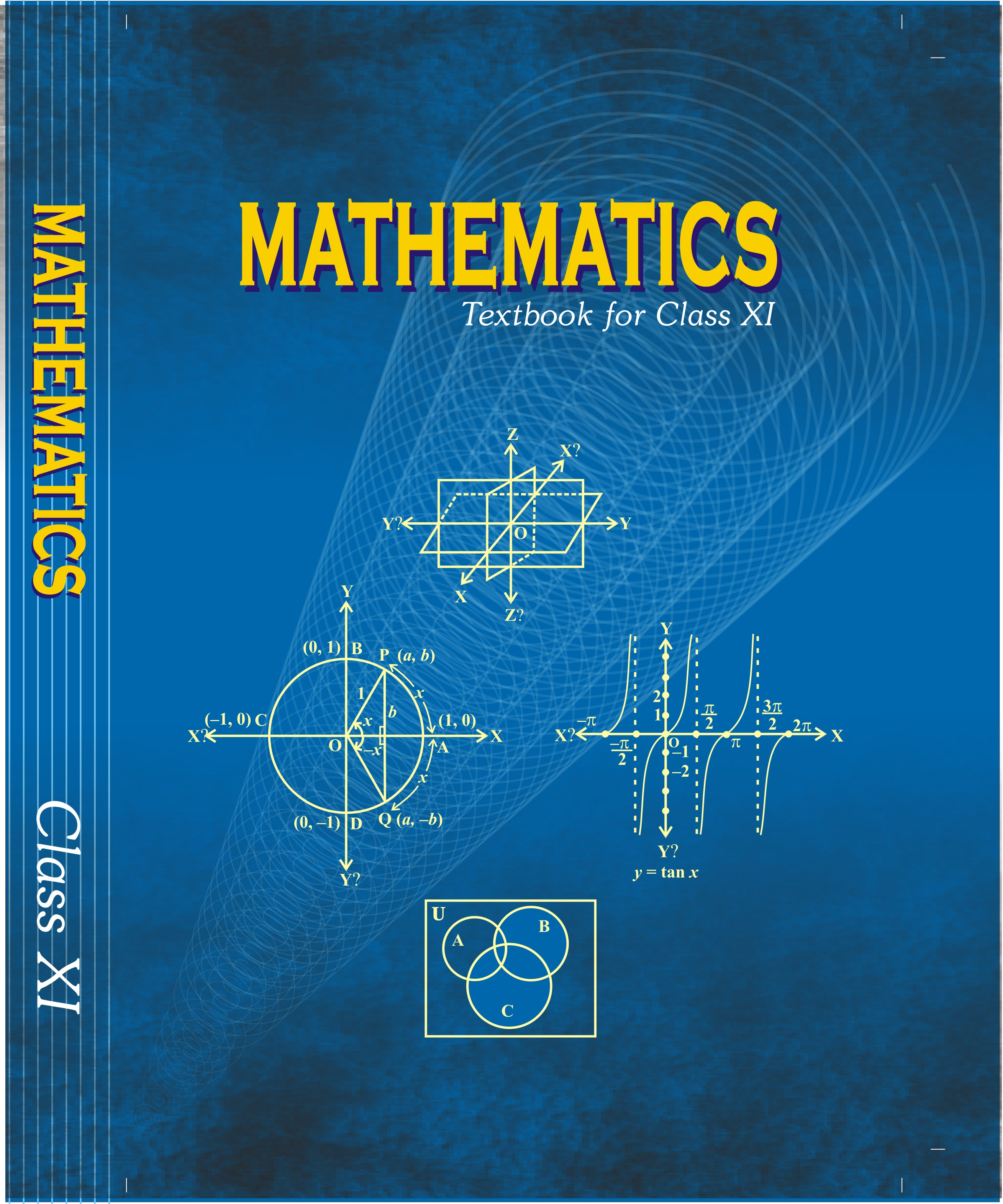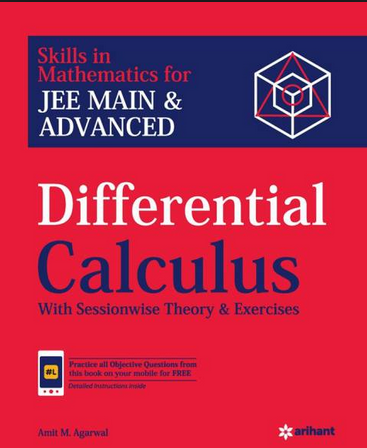# Limit , continuity and differentiability   Share

## What is Limit , continuity and differentiability

In Mathematics, Limits continuity and differentiability act as a building block for the whole calculus. So by being the basic topic for calculus, it becomes a very important topic to be understood, Questions of this chapter has lots of variation as the chapter itself has 3 independent topics so it becomes a large chapter too and hence provide variations in the type of questions, level of questions. Every year you will get at max 2 - 4 questions in JEE Main and other exams, directly (as chapter weight in jee main is only 10%) but indirectly, this chapter is going to serve as building blocks for the entire calculus. In area finding problem we first see if the function is continuous or not, so in that way, it will provide some handy tool for various other types of problems and chapters.

It will be a new chapter for student and you will find it difficult to learn initially but with time once you understand the basic concept and do a proper amount of practice you will find it handy. This chapter is highly calculative, so you need to do proper practice, you should solve an ample amount of questions and make sure you are good with calculations. Overall this chapter will be larger than other chapters as it has three independent topics combined.

## Why Limit, Continuity, and Differentiability:

Limits, continuity, and differentiability are important for being the building blocks of whole calculus. The various that you will study in this chapter are itself very useful in various field life in physics finding the electric field, magnetic field, gravitational force, finding the area, force and so on. The area finding an application of continuity function is used to by architect-engineer to determine the need for material for the construction. If you understand this chapter well you will realize how good tools this chapter provides to you. You will love to solve questions based on this chapter.

Prepare Online for JEE Main/NEET

Crack JEE 2021 with JEE/NEET Online Preparation Program

After studying Limit, continuity, and differentiability:

1. After studying this chapter you will find new tools of mathematics which you will be exploring, and maybe you will enjoy using it.

2. You will feel comfortable with the calculative problem of mechanics and electro physics in physics which involves the concept of this chapter.

3. This chapter will give you a different approach of thinking to solve a problem in physics and in general in academic research which will make you more equipped to deal with the problem and hence confident.

4. And obviously, the chapter itself will help you to score some marks in the exam as it gets about 10% weight in jee main.

## Notes on Limit, Continuity, and Differentiability:

Important topics :

1. Limits of a function, properties of limits

2. Limits of polynomials and rational functions

3. Continuity of a function at a point

4. Discontinuity

5. Continuity of composite functions

6. Differentiability

7. Algebra of derivatives

8. Rolle’s Theorem

9. Mean Value Theorem (Lagrange)

## Overview of the Limit, Continuity, and Differentiability:

Limits of function: Let f be a function defined in a domain which we take to be an interval, say, I. We shall study the concept of limit of f at a point ‘a’ in I.

We say lim f(x) is the expected value of f at x = a given the values of f near the x→a- left of a. This value is called the left-hand limit of f at a.

We say lim f(x) is the expected value of f at x = a given the values of f near to the

X→a+ right of a. This value is called the right-hand limit of f at a.

If the right and left hand limits coincide, we call the common value as the limit of f at x = a and denote it by .

Let f and g be two functions such that both   exist. Then

Limits of the polynomial:  If f is a polynomial function, then  exists and is given by

Continuity of a function at a point:

Let f be a real function on a subset of the real numbers and let c be a point in the domain of f. Then f is continuous at c if

lim

or, if the left-hand limit, right-hand limit and the value of the function at x = c exist and are equal to each other, i.e.,

Discontinuity: The function f will be discontinuous at x = a in any of the following cases :

1.  exist but are not equal
2.     exist and are equal but not equal to f (a)
3. f (a) is not defined.

Continuity of composite function: Let f and g be real-valued functions such that (fog) is defined at a. If g is continuous at a and f is continuous at g (a), then (fog) is continuous at a.

## How to prepare Limits, Continuity, and Differentiability:

Limits, continuity, and differentiability is a very important topic to be understood.  This is the basic building block of entire calculus and it is used as a tool in physics, so you must be through with this chapter.

1. To understand this topic you have to go in the order of the name of the topic of this chapter means first you must study about what is the limit and its type then notation of continuity and then basic integration.

2. Start with understanding basic concepts like simple definitions (what is limit, what is continuity and definition of differentiability), understand each topic independently before moving to another by going through every concept.

3. Once you’re clear with basic concepts move to complex concepts, like limits of algebraic functions, continuity of a function at a given point and it’s differentiability at the same point.

4. After studying these concepts go through solved examples and then go to mcq and practice the problem to make sure you understood the topic.

5.  Solve the questions of the books which you are following and then go to previous year papers.

6. While going through concept make sure you understand the derivation of formulas and try to derive them by your own, as many times you will not need the exact formula but some steps of derivation will be very helpful to solve the problem if you understand the derivation it will boost your speed in problem-solving.

7. At the end of chapter try to make your own short notes for quick revision, make a list of formula to revise quickly before exams or anytime when you required to revise the chapter, it will save lots of time for you.

## Best books for the preparation Limits, Continuity, and Differentiability:

First, finish all the concept, example and questions given in NCERT Maths Book. You must be thorough with the theory of NCERT. Then you can refer to the book Limit, continuity and differentiability (Arihant) by Amit m. Aggarwal or RD Sharma but make sure you follow any one of these not all. differential calculus is explained very well in these books and there are an ample amount of questions with crystal clear concepts. Choice of reference book depends on person to person, find the book that best suits you the best, depending on how well you are clear with the concepts and the difficulty of the questions you require.

## Maths Chapter-wise Notes for Engineering exams

 Chapters Chapters Name Chapter 1 Sets, Relations, and Functions Chapter 2 Complex Numbers and Quadratic Equations Chapter 3 Matrices and Determinants Chapter 4 Permutations and Combinations Chapter 5 Binomial Theorem and its Simple Applications Chapter 6 Sequence and Series Chapter 8 Integral Calculus Chapter 9 Differential Equations Chapter 10 Coordinate Geometry Chapter 11 Three Dimensional Geometry Chapter 12 Vector Algebra Chapter 13 Statistics and Probability Chapter 14 Trigonometry Chapter 15 Mathematical Reasoning Chapter 16 Mathematical Induction

### Topics from Limit , continuity and differentiability

• Graphs of simple functions, limits, continuty and differentiability. ( JEE Main, KCET, SRMJEEE, MET, MHT-CET, KEAM ) (55 concepts)
• Differentiation of the sum, difference, product and quotient of two functions ( JEE Main, COMEDK UGET, KEAM ) (16 concepts)
• Differentiation of trigonometric, inverse trigonometric, logarithmic exponential, composite and implicit functions ( JEE Main, COMEDK UGET, KEAM ) (16 concepts)
• Derivatives of order upto two ( JEE Main, COMEDK UGET, KEAM ) (1 concepts)
• Apllications of derivatives monotonic, maxima and minima of functions of one variable, tangents and normals ( JEE Main, COMEDK UGET, KEAM ) (34 concepts)
• Monotonic- increasing and decreasing functions ( JEE Main, COMEDK UGET, KEAM ) (8 concepts)
• Rolle's and Lagrange's Mean Value theorems ( JEE Main, COMEDK UGET, KEAM ) (5 concepts)
• Limits and their Importance ( JEE Main, COMEDK UGET, KEAM ) (17 concepts)
• Differentiation ( JEE Main, COMEDK UGET, KEAM ) (17 concepts)
• Continuity and Differentiability ( JEE Main, COMEDK UGET, KEAM ) (13 concepts)
• Application of Derivatives ( JEE Main, COMEDK UGET, KEAM ) (8 concepts)
• Monotonicity and Maxima-Minima of Functions ( JEE Main, COMEDK UGET, KEAM ) (14 concepts)

### Important Books for Limit , continuity and differentiability

•••Exams
Articles
Questions